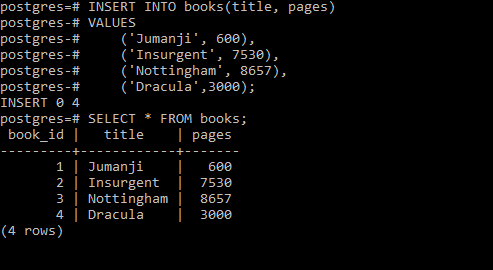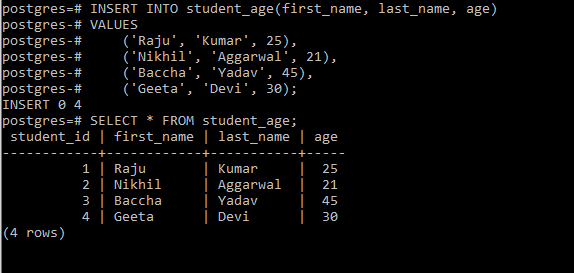# PostgreSQL – SMALLINT Integer Data Type

• Last Updated : 28 Aug, 2020

PostgreSQL allows a type of integer type namely `SMALLINT`. It requires 2 bytes of storage size and can store integers in the range of -37, 767 to 32, 767. It comes in handy for storing data like the age of people, the number of pages in a book, etc.

`Syntax: variable_name SMALLINT`

Now let’s look into some examples of use cases of SMALLINT integer type.
Example 1:
In this example we will create a table that stores the number of pages in a book by using the below commands:

```CREATE TABLE books (
book_id SERIAL PRIMARY KEY,
title VARCHAR (255) NOT NULL,
pages SMALLINT NOT NULL CHECK (pages > 0)
);```

Now let’s add some data to the table using the below command:

```INSERT INTO books(title, pages)
VALUES
('Jumanji', 600),
('Insurgent', 7530),
('Nottingham', 8657),
('Dracula', 3000);```

Now let’s check our inserted data using the below commands:

`SELECT * FROM books;`

Output:Example 2:
In this example we will create a table that stores the ages of students by using the below commands:

```CREATE TABLE student_age(
student_id SERIAL PRIMARY KEY,
first_name VARCHAR (255) NOT NULL,
last_name VARCHAR (255) NOT NULL,
age SMALLINT NOT NULL CHECK (age > 0)
);```

Now let’s add some data to the table using the below command:

```INSERT INTO student_age(first_name, last_name, age)
VALUES
('Raju', 'Kumar', 25),
('Nikhil', 'Aggarwal', 21),
`SELECT * FROM student_age;`